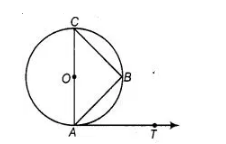# If AB is a chord of a circle with centre 0,

Question:

If AB is a chord of a circle with centre 0, AOC is a diameter and AT is the tangent at A as shown in figure. Prove that ∠BAT = ∠ACB.Solution:

Since, AC is a diameter line, so angle in semi-circle makes an angle 90°.

$\begin{array}{lll}\therefore & \angle A B C=90^{\circ} & \text { [by property] }\end{array}$

In $\triangle A B C, \quad \angle C A B+\angle A B C+\angle A C B=180^{\circ}$

$\left[\because\right.$ sum of all interior angles of any triangle is $\left.180^{\circ}\right]$

$\Rightarrow \quad \angle C A B+\angle A C B=180^{\circ}-90^{\circ}=90^{\circ}$ $\ldots($ (i)

Since, diameter of a circle is perpendicular to the tangent.

i.e. $C A \perp A T$

$\therefore \quad \angle C A T=90^{\circ}$

$\Rightarrow \quad \angle C A B+\angle B A T=90^{\circ} \quad \ldots$ (ii)

From Eqs. (i) and (ii),

$\angle C A B+\angle A C B=\angle C A B+\angle B A T$

$\Rightarrow \quad \angle A C B=\angle B A T \quad$ Hence proved.